Test: Recurrence & Searching- 2

# Test: Recurrence & Searching- 2

Test Description

## 15 Questions MCQ Test GATE Computer Science Engineering(CSE) 2023 Mock Test Series | Test: Recurrence & Searching- 2

Test: Recurrence & Searching- 2 for Computer Science Engineering (CSE) 2023 is part of GATE Computer Science Engineering(CSE) 2023 Mock Test Series preparation. The Test: Recurrence & Searching- 2 questions and answers have been prepared according to the Computer Science Engineering (CSE) exam syllabus.The Test: Recurrence & Searching- 2 MCQs are made for Computer Science Engineering (CSE) 2023 Exam. Find important definitions, questions, notes, meanings, examples, exercises, MCQs and online tests for Test: Recurrence & Searching- 2 below.
Solutions of Test: Recurrence & Searching- 2 questions in English are available as part of our GATE Computer Science Engineering(CSE) 2023 Mock Test Series for Computer Science Engineering (CSE) & Test: Recurrence & Searching- 2 solutions in Hindi for GATE Computer Science Engineering(CSE) 2023 Mock Test Series course. Download more important topics, notes, lectures and mock test series for Computer Science Engineering (CSE) Exam by signing up for free. Attempt Test: Recurrence & Searching- 2 | 15 questions in 45 minutes | Mock test for Computer Science Engineering (CSE) preparation | Free important questions MCQ to study GATE Computer Science Engineering(CSE) 2023 Mock Test Series for Computer Science Engineering (CSE) Exam | Download free PDF with solutions
 1 Crore+ students have signed up on EduRev. Have you?
Test: Recurrence & Searching- 2 - Question 1

### Consider the following recurrence: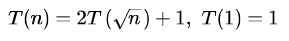Which one of the following is true?

Detailed Solution for Test: Recurrence & Searching- 2 - Question 1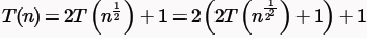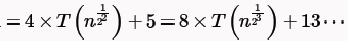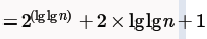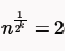( Putting 2so that we can take log. one more step of recurrence can't change the complexcity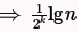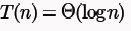Test: Recurrence & Searching- 2 - Question 2

### Let Xn denote the number of binary strings of length n that contain no consecutive 0s. Which of the following recurrences does Xn satisfy?

Detailed Solution for Test: Recurrence & Searching- 2 - Question 2

0 1 -2
01 10 11 -3
010 011 101 110 111 -5
0101 0110 0111 1010 1011 1101 1110 1111 -8

So, xn = xn-1 + xn-2 (For all the strings ending in 1, we get two new strings and for all strings ending in 0, we get a new string. So, the new set of strings for n+1, will have exactly n strings ending in 1)

x5 = 8+5 = 13

Test: Recurrence & Searching- 2 - Question 3

### Let Xn denote the number of binary strings of length n that contain no consecutive 0s. x5 = 8+5 = 13

Detailed Solution for Test: Recurrence & Searching- 2 - Question 3

Number of binary strings of length n that contain no consecutive 0s, following will be the required recurrence relation:

T(n) = T(n-1) + T(n-2) n>2

base condition T(1) = 2 and T(2) = 3

T(1) =2 There will be 2 strings of length 1, i.e 0 & 1
T(2) =3 There will be 3 strings of length 2, i.e. 01,10,11

T(3) = T(1) + T(2) = 2+3 = 5
T(4) = T(3) + T(2) = 5 + 3 = 8
T(5) = T(4) +T(3) = 8 + 5 = 13

Test: Recurrence & Searching- 2 - Question 4

When n = 22k for some  k ≥ 0, the recurrence relation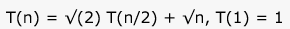evaluates to :

Detailed Solution for Test: Recurrence & Searching- 2 - Question 4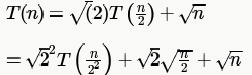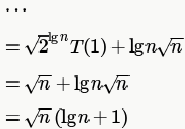If we use Master theorem we get option B. But one must know that Matser theorem is used to find the asymptotic bound and not an EXACT value. And in the question here it explicitly says "evaluates to".

Test: Recurrence & Searching- 2 - Question 5

The running time of an algorithm is represented by the following recurrence relation: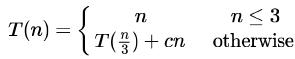Which one of the following represents the time complexity of the algorithm?

Detailed Solution for Test: Recurrence & Searching- 2 - Question 5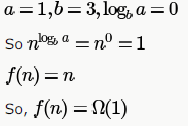To, check Master theorem case 3, we need C>0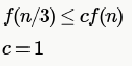So using case three of master theorem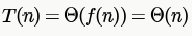Test: Recurrence & Searching- 2 - Question 6

The recurrence relation capturing the optimal execution time of the Towers of Hanoi  problem with n discs is

Detailed Solution for Test: Recurrence & Searching- 2 - Question 6

Recurrence relation for Towers of Hanoi is
T(1) = 1
T(n) = 2 T( n-1 ) +1

Test: Recurrence & Searching- 2 - Question 7

Which one of the following correctly determines the solution of the recurrence relation with T(1) =1?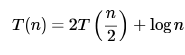Detailed Solution for Test: Recurrence & Searching- 2 - Question 7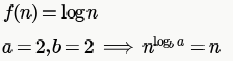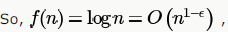we can take any ε from 0-1 for example 0.5 which gives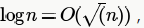whose proof
is given here: http://math.stackexchange.com/questions/145739/prove-that-logn-o-sqrtn

Test: Recurrence & Searching- 2 - Question 8

Let an represent the number of bit strings of length n containing two consecutive 1s. What is the recurrence relation for an ?

Detailed Solution for Test: Recurrence & Searching- 2 - Question 8

Counting the number of bit strings NOT containing two consecutive 1's. (It is easy to derive a recurrence relation for the NOT case as shown below.)

0 1
00 01 10 - 3 (append both 0 and 1 to any string ending in 0, and append 0 to any string ending in 1)

000 001 010 100 101 - 5 (all strings ending in 0 give two strings and those ending in 1 give 1 string)

0000 0001 0010 0100 0101 1000 1001 1010 - 8

....

an' = an-1' + an-2' (where an denote the number of bit strings of length n containing two consecutive 1s)

2n - an = (2n-1 - an-1) + (2n-2 - an-2)

an = 2n-2(4 - 2 - 1) + an-1 + an-2

an = an-1 + an-2 + 2n-2

Test: Recurrence & Searching- 2 - Question 9

Consider the following recursive C function.

void get(int n)

{
if (n<1) return;

get (n-1);

get (n-3);

printf("%d", n);

}

If get(6) function is being called in main() then how many times will the get() function be invoked before returning to the main()?

Detailed Solution for Test: Recurrence & Searching- 2 - Question 9

T(n) = T(n-1) + T(n-3) + 2, here T(n) denotes the number of times a recursive call is made for input n. 2 denotes the two direct recursive calls.

T(n<=0) = 0
T(1) = 2
T(2) = 4
T(3) = 6
T(4) = 10
T(5) = 16
T(6) = 24

So, answer is 24 + 1 call from main = 25.

Test: Recurrence & Searching- 2 - Question 10

Consider the recurrence function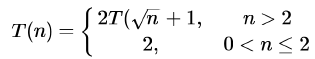Then T(n) in terms of θ notation is

Detailed Solution for Test: Recurrence & Searching- 2 - Question 10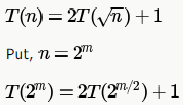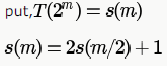Using case 1 of master method ,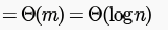Test: Recurrence & Searching- 2 - Question 11

Consider the following recurrence relation: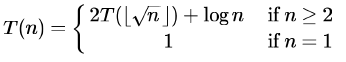Which of the following statements is TRUE?

Detailed Solution for Test: Recurrence & Searching- 2 - Question 11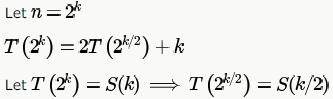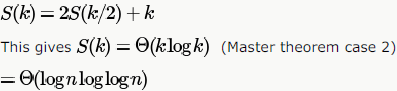Test: Recurrence & Searching- 2 - Question 12

The average number of key comparisons required for a successful search for sequential search on n items is

Detailed Solution for Test: Recurrence & Searching- 2 - Question 12

Expected number of comparisons

= 1x Probability of first element be x + 2 x Probability of second element be x + .... +n x Probability of last element be x.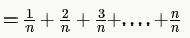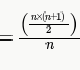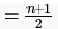Test: Recurrence & Searching- 2 - Question 13

Consider the following C program that attempts to locate an element x in an array Y[ ] using binary search. The program is erroneous.

f (int Y , int x) {

int u, j, k;

i= 0; j = 9;

do {

k = (i+ j) / 2;

if( Y[k] < x) i = k; else j = k;

} while (Y[k] != x) && (i < j)) ;

if(Y[k] == x) printf(" x is in the array ") ;

else printf(" x is not in the array ") ;

}

On which of the following contents of Y and x does the program fail?

Detailed Solution for Test: Recurrence & Searching- 2 - Question 13

when it is option C the control will continue to iterate as i=8 and j=9;

again and again i will be assigned k which itself equals 8 as 8+9/2 being stored in an integer type variable, will evaluate to 8

For option A, with X=9 , k will take the following values:

• 4
• 6
• 7
• 8 - y = 9, x found

For option D, with X = 10, k will take the following values:

• 4, y = 10, x found
Test: Recurrence & Searching- 2 - Question 14

Consider the following C program that attempts to locate an element x in an array Y[ ] using binary search. The program is erroneous.

f (int Y , int x) {

int u, j, k;

i= 0; j = 9;

do {

k = (i+ j) / 2;

if( Y[k] < x) i = k;else j = k;

} while (Y[k] != x) && (i < j)) ;

if(Y[k] == x) printf(" x is in the array ") ;

else printf(" x is not in the array ") ;

}

The correction needed in the program to make it work properly is

Detailed Solution for Test: Recurrence & Searching- 2 - Question 14

if( Y[k] < x) then i = k + 1;

if given element that we are searching is greater then searching will be continued upper half of array

otherwise j = k - 1;
lower half.
Take few case in consideration i.e.

1. all elements are same
2. increasing order with no repeatation
3. increasing order with repeatation.

Test: Recurrence & Searching- 2 - Question 15

Suppose you are given an array A with 2n numbers.

The numbers in odd positions are sorted in ascending order, that is,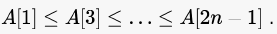The numbers in even positions are sorted in descending order, that is,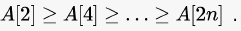What is the method you would recommend for determining if a given number is in the array?

Detailed Solution for Test: Recurrence & Searching- 2 - Question 15

We can simply use clever indexing to binary search the element in the odd positions, and in the even positions separately.

This will take O(logn) time andO(1) space in the worst case.

A: Sorting using Quicksort will take O(n2) time.

B: Merging will take O(n) time and O(n) space.

C: Binary search only works on a sorted array.

E: Sequential search will take O(n) time.

## GATE Computer Science Engineering(CSE) 2023 Mock Test Series

150 docs|215 tests
 Use Code STAYHOME200 and get INR 200 additional OFF Use Coupon Code
Information about Test: Recurrence & Searching- 2 Page
In this test you can find the Exam questions for Test: Recurrence & Searching- 2 solved & explained in the simplest way possible. Besides giving Questions and answers for Test: Recurrence & Searching- 2, EduRev gives you an ample number of Online tests for practice

## GATE Computer Science Engineering(CSE) 2023 Mock Test Series

150 docs|215 tests